Mobile QR Code1. (Chung-Ang University, Seoul 06974, Korea )

Clock generator, phase-locked loop (PLL), low jitter, phase noise, multiplying delay locked loop (MDLL), sub-sampling PLL (SSPLL), reference-sampling PLL (RSPLL)

## I. INTRODUCTION

As mobile smart device technology continues to develop, high-performance, multi-functional audio chips have become increasingly required. The audio system presented in Fig. 1 converts signals using analog-to-digital converters (ADCs) or digital-to-analog converters (DACs) to transmit and receive various types of audio sources. The phase-locked loop (PLL) or clock manager provides the clock frequencies for each block, which has a significant effect on the performance of the audio system. Recent digital audio systems typically employ the frequencies of 12.288, 24.576, and 49.152 MHz. An Integrated Interchip Sound (I$^{2}$S) standard uses frequency of 768 kHz to 12.288 MHz, which is also used for reference clock in PLLs .

One of the important performance indexes for a PLL is RMS jitter. The effect of jitter in the sampling process is shown in Fig. 2. A sampling clock with jitter results in errors between the sampled and original data, which causes sound quality degradation. A low jitter PLL is required for high-performance audio systems. We implement, measure, and compare PLLs of various structures currently being studied for low jitter performance. The charge-pump-based integer-N PLL (NPLL) structure presented in Fig. 3(a) has been widely adopted. The N-divider in the feedback loop leads to N$^{2}$ multiplication of the phase noises from noisy components, making it difficult to make a low jitter PLL . To overcome this limitation, various PLL configurations have been studied such as multiplying delay-locked loops (MDLLs), sampling-based PLLs, and digital PLLs [3-6].

In this paper, two tri-state phase frequency detector (PFD)-based PLLs (an NPLL and MDLL) and two sampling-based PLLs (a sub-sampling PLL [SSPLL] and reference-sampling PLL [RSPLL]) with the same supply voltage, reference frequency, and voltage-controlled oscillator (VCO) are studied. The noise characteristics of the PLLs are first compared theoretically, then the PLLs are fabricated on a silicon die for measurements.

##### Fig. 1. Block diagram of an audio system with a PLL and clock manager.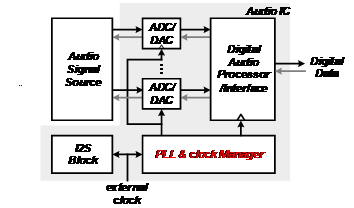##### Fig. 2. Sampling process with the jitter effect.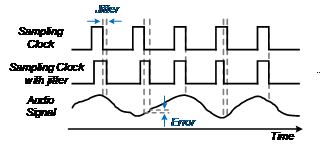##### Fig. 3. (a) Block diagram of the NPLL core block; (b) timing diagram.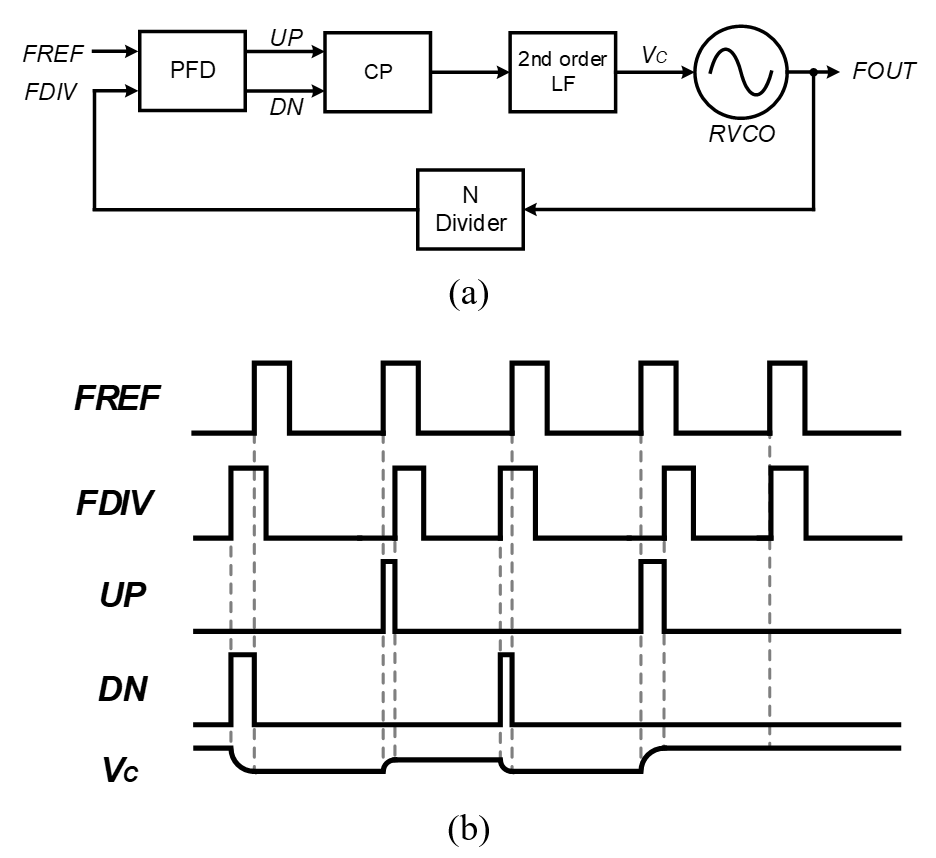## II. PLL CONFIGURATIONS FOR AUDIO SYSTEMS

The structures of the audio PLLs are classified into two parts: Tri-state PFD-based PLL and sampling-based PLL. These audio PLLs are composed of the core block, pre-divider, and post-divider.

Fig. 4(a) is a block diagram of an MDLL, which is a tri-state PFD-based PLL. The jitter performance of the MDLL is improved by applying a reference edge to the ring VCO (RVCO) output signal every reference period to remove the accumulated jitter . However, this periodic operation causes undesired reference spur in the frequency domain, which appears as jitter in the time domain.

Sampling-based PLLs such as SSPLL and RSPLL achieve low jitter for a given power consumption [8-12]. As shown in Fig. 5(a) and 6(a), the SSPLL samples the reference signal (FREF) with the RVCO output signal (FOUT), and the RSPLL samples the FOUT with the FREF [13,14]. The SSPLL can drastically reduce jitter by eliminating the N$^{2}$ multiplication. However, unlike the SSPLL, the loop gain for the RSPLL contains a virtual division by N, thus it cannot drastically reduce jitter by eliminating N$^{2}$ multiplication. However, the high gain obtained by the sampling phase detector (SPD) used in sampling-based PLLs can reduce the noise from subsequent blocks.

##### Fig. 4. (a) Block diagram of the MDLL core block; (b) timing diagram.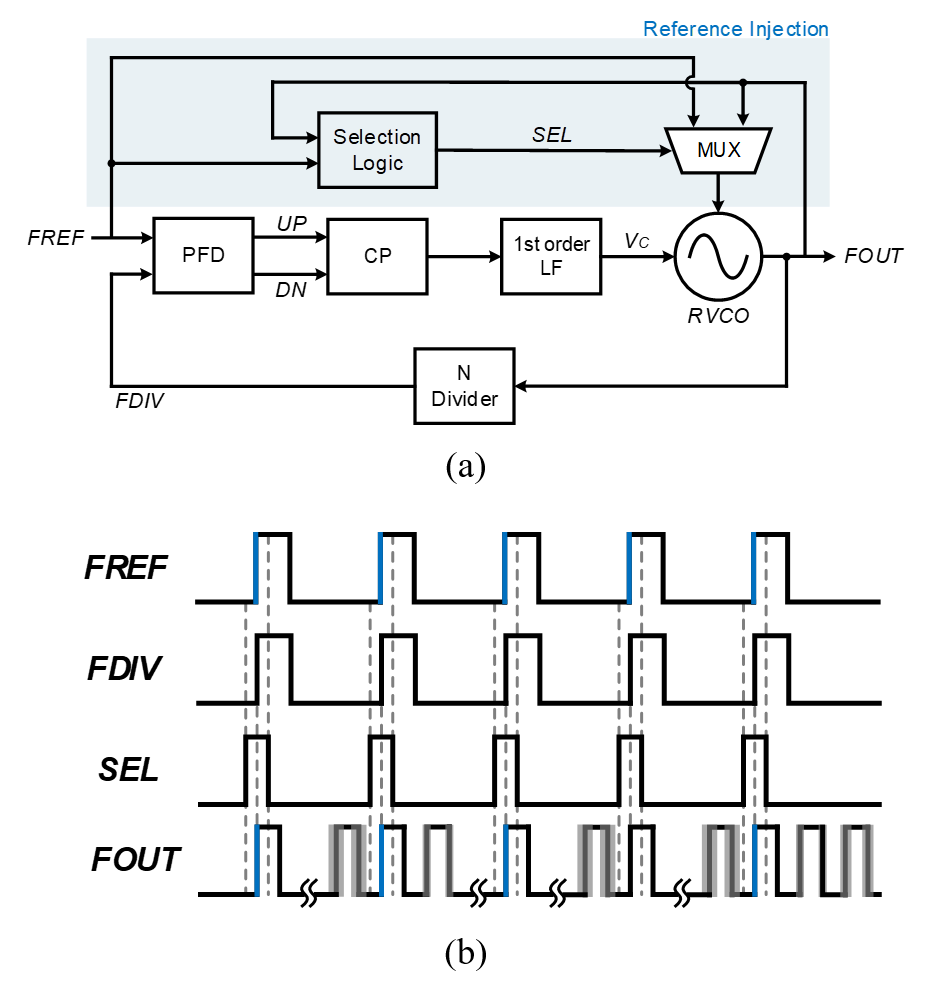##### Fig. 5. (a) Block diagram of the SSPLL core block; (b) timing diagram.##### Fig. 6. (a) Block diagram of the RSPLL core block; (b) timing diagram.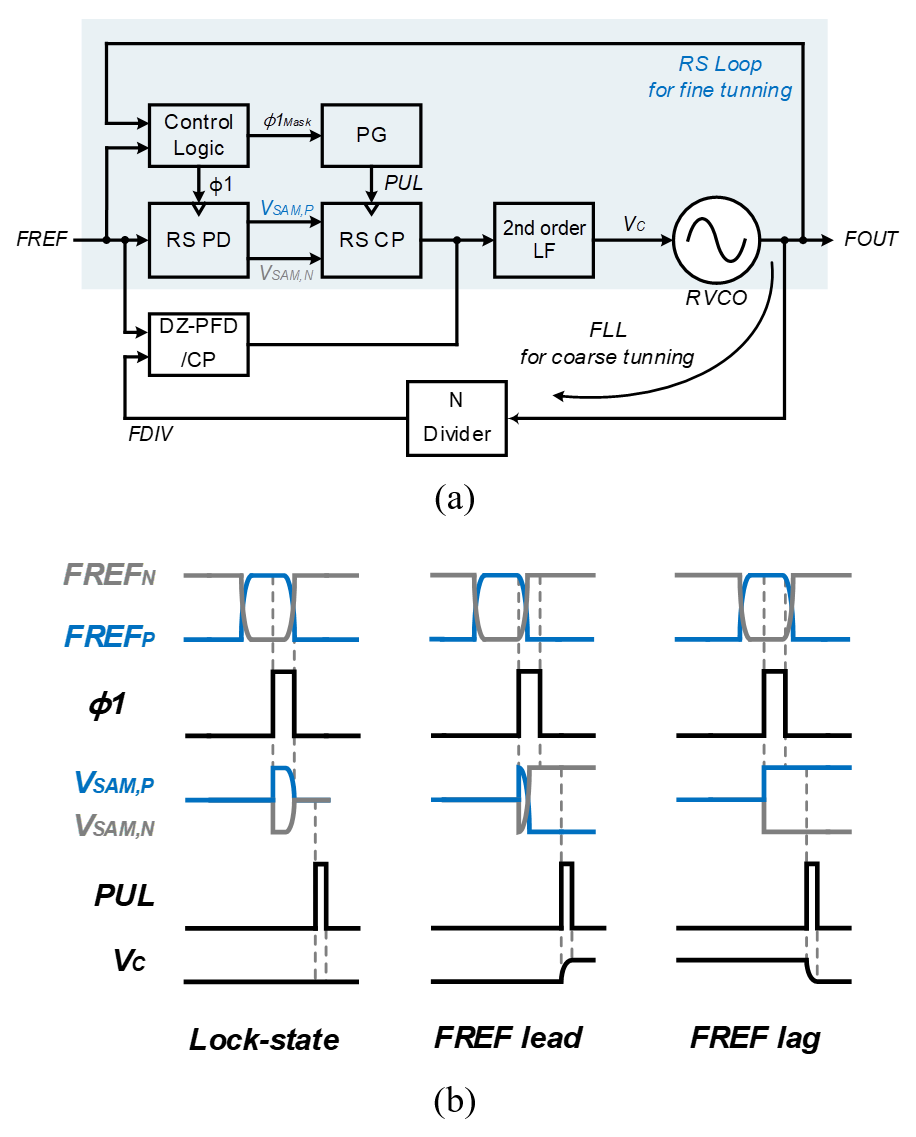### 1. Tri-state PFD-based PLLs

A tri-state PFD-based PLL detects the phase difference between the FREF, and N-divider output signal (FDIV), and outputs it as the width of the UP/DN pulse. An NPLL consists of a tri-state PFD, a charge pump (CP), a second-order loop filter (LF), an RVCO, and an N-divider (Fig. 3(a)). The current of the CP is proportional to the width of the UP/DN pulse. The CP controls V$_{C}$ and determines the frequency of the RVCO output, which is fed back through the N-divider to the PFD.

Fig. 3(b) presents a timing diagram of an NPLL. When the FDIV lags, a UP pulse is generated by the PFD and the CP current flows into the LF. As V$_{C}$ increases, the FOUT frequency also increases. When the FDIV leads, a DN pulse is generated by the PFD, and the sink current from the LF slows FOUT.

As shown in Fig. 4(a), an MDLL consists of a tri-state PFD, a CP, a first-order LF, an RVCO, a multiplexer, selection logic, and an N-divider. Inputs of the selection logic are the FOUT and the FREF. The selection logic generates the selection signal (SEL) for the multiplexer. When SEL is HIGH, the multiplexer replaces an edge of FOUT with an FREF edge every reference period. When SEL is low, the MDLL operates in the same manner as an NPLL. Fig. 4(b) shows a timing diagram for when the MDLL is in a locked state.

### 2. Sampling-based PLLs

As presented in Fig. 5(a) and 6(a), sampling-based PLLs have dual control loops. Initially, both the coarse and fine loops operate. The coarse loop is used for fast frequency acquisition. After frequency acquisition, the operation of the coarse loop stops, and the fine loop controls the VCO only. For dual-loop operation, the PFD is modified to have a dead zone (DZ). If the phase difference between the two input signals for the DZ-PFD is less than a specified DZ, the output is not produced by the DZ-PFD. The SPD then detects the phase difference between FREF and FOUT.

Fig. 5(a) is a block diagram for an SSPLL. The coarse loop consists of a DZ-PFD, a CP, a second-order LF, an RVCO, and an N-divider. The fine loop uses a sub-sampling phase detector (SSPD) and a sub-sampling CP (SSCP). A pulse generator (PG) is added for SSCP operation, while an N-divider is not included in the fine loop. The SSPD has a differential structure that samples differential FOUT simultaneously. The difference between differentially sampled values (V$_{SAM,P}$ and V$_{SAM,N}$) determines the SSCP current.

A timing diagram for the SSPLL is shown in Fig. 5(b). In a locked state, the output of the SSPD is ideally constant at half of the input signal amplitude (A$_{INPUT}$/2), which is the crossing point of the differential input signal. However, when FREF lags, the output of V$_{SAM,N}$ is larger than A$_{INPUT}$/2 and the sink current from the V$_{C}$ node produces a lower V$_{C}$ and the FOUT frequency. In contrast, when FREF leads, the output of V$_{SAM,N}$ is smaller than A$_{INPUT}$/2 and the source current from the V$_{C}$ node produces a higher V$_{C}$ and the FOUT frequency. When the SSPD outputs are same, the output current of SSCP is zero. The SSCP affects V$_{C}$ node only when PUL is HIGH.

As shown in Fig. 5(a) and 6(a), the differences between the SSPLL and RSPLL originate from the fine loop. The sampling directions of the reference-sampling phase detector (RSPD) and SSPD oppose each other. In the SSPLL, the SSPD can detect phase differences at the edge of FREF without additional circuits. However, in the RSPLL, an additional control block is required to compare phases near the edge of FREF because the RSPD samples FREF by FOUT, which is N times faster than FREF. The control logic generates a sampling clock synchronized with FOUT. After sampling the signal in the RSPD, V$_{C}$ is controlled by the output current from reference-sampling CP (RSCP), whose schematic is the same as the SSCP. However, because of the opposing sampling directions, the effects of V$_{SAM,P}$ and V$_{SAM,N}$ on V$_{C}$ are also the opposite.

A timing diagram of an RSPLL is shown in Fig. 6(b). If V$_{SAM,P}$ is higher than V$_{SAM,N}$, the sink current from the V$_{C}$ node reduces the frequency of the RVCO and, if V$_{SAM,P}$ is lower than V$_{SAM,N}$, the source current to the V$_{C}$ node increases the frequency. The RSCP only affects V$_{C}$ node when PUL is HIGH.

Fig. 7(a) shows the phase-to-current gain characteristics of the SSPD/SSCP in an SSPLL without a coarse loop (i.e., an FLL loop). The SSPLL has a narrow lock range of T$_{RVCO}$/2. If a coarse loop is added, it follows the phase-to-current gain characteristics of a general PFD/CP outside the DZ. Because the loop gain of the coarse loop is higher than that of the fine loop, the coarse loop mainly shifts the phase of the VCO into the lock range when a phase error higher than the dead zone occurs. Fig. 7(c) is the gain characteristic of an RSPD/RSCP without a coarse loop. The proposed RSPLL is designed to maximize the RSPD gain near the lock point to reduce the CP current and minimize the phase noise. Even though the RSPLL has a wide lock range of T$_{REF}$, a coarse loop is required to reduce the locking time by adding a DZ-PFD and CP (Fig. 7(d)) .

##### Fig. 7. Phase-to-current gain characteristics for (a) an SSPLL without; (b) with a DZ-PFD/CP; (c) an RSPLL without; (d) with a DZ-PFD/CP.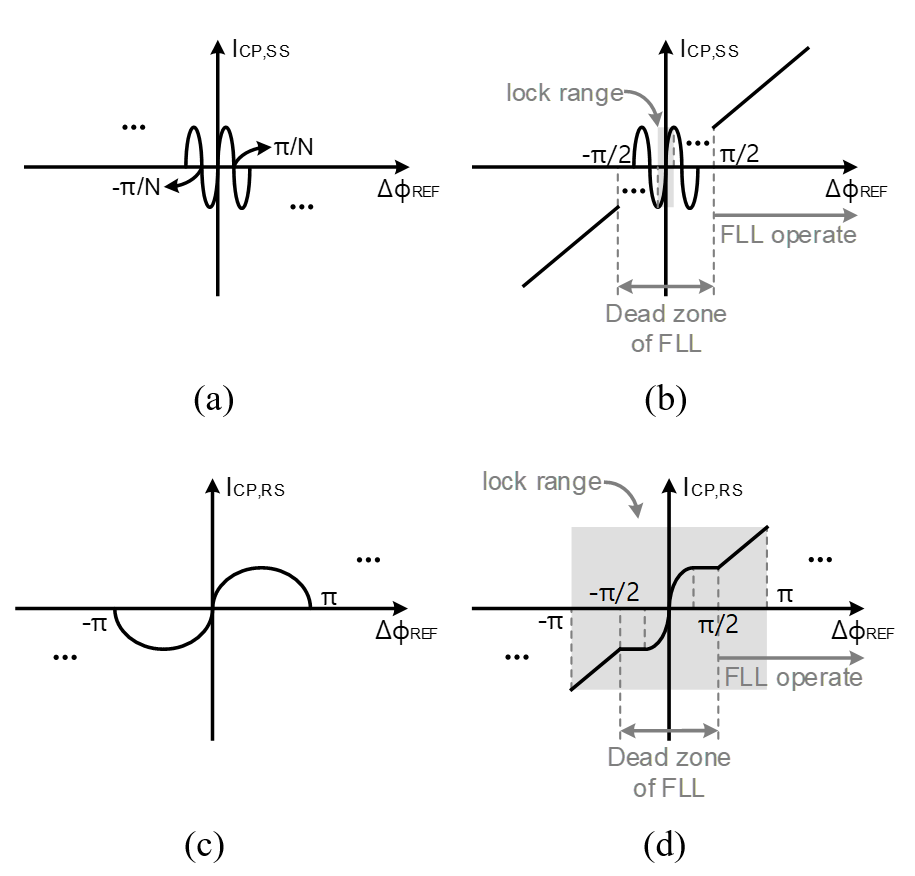## III. CIRCUIT DESCRIPTION

### 1. Tri-state PFD-based PLLs

The NPLL and MDLL in the present study use a tri-state PFD to compare the frequency and phase of FREF and FDIV. The tri-state PFD in Fig. 8 consists of two D-flip flops and an AND gate. When the frequency of FREF is higher than that of FDIV, an UP pulse is generated by the PFD. When the frequency of FDIV is higher than that of FREF, a DN pulse is generated. The CP has a constant UP/DN current (I$_{UP}$/I$_{DN}$). The UP pulse produces a current source in proportion to I$_{UP}$ and the width of the UP and DN pulses induces a current source, sinking the current in proportion to I$_{DN}$ and the DN pulse width. When the PLL is locked, the Q of the two D-flip-flops generates identical pulses. Thus, the charge pump current becomes zero and it no longer affects the LF output.

##### Fig. 8. Block diagram for a DZ-PFD.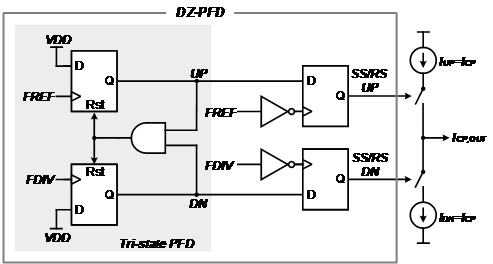### 2. Sampling-based PLLs

The coarse loops of the SSPLL and RSPLL use a DZ-PFD, which consists of four D-flip flops. The DZ in the DZ-PFD in Fig. 8 is T$_{REF}$/2 because inverted FREF enters the clock of the two D-flip flops following the tri-state PFD. The DZ-PFD does not generate a UP/DN pulse with a UP/DN pulse width smaller than T$_{REF}$/2. The operation of the coarse loop thus does not affect the loop.

An SPD is presented in Fig. 9. The SSPLL and RSPLL use an SPD in their fine loop operation. Unlike a tri-state PFD, it is easy to control the gain, and it has the advantage of being able to produce a large gain. The SPD consists of eight transmission gates and has a symmetric structure. The dummies in the SPD are for constant input impedance. The SSPD and RSPD sample the differential input and convert the phase difference into a voltage difference.

The UP/DN currents of the SSCP and RSCP are altered by V$_{IN,P}$ and V$_{IN,N}$ (Fig. 10). In particular, 2${\cdot}$I$_{B}$ is divided into M1 and M2 according to the difference between V$_{IN,P}$ and V$_{IN,N}$. The current for M2 becomes the down current for the output stage. I$_{CP,OUT}$ is determined by subtracting the fixed I$_{B}$ current (I$_{UP}$) and I$_{M2}$ (I$_{DN}$). When the PLL is locked, I$_{CP,OUT}$ is zero because V$_{SAM,P}$ and V$_{SAM,N}$ are equal (the UP/DN currents are equal). Transconductance g$_{m}$ originates from the single MOS transistor in M1 and M2. I$_{CP,OUT}$ affects the next block only when the PUL signal is HIGH.

##### Fig. 10. Schematic of a sampling-based CP.### 3. Ring-VCO

The four PLLs in the present study employ the same RVCO. A schematic of a four-stage fully differential RVCO is shown in Fig. 11. A differential VCO can provide better common mode supply rejection and ground noise suppression. An RVCO is designed to provide wide-range frequency signals and has the advantage of a small area . The differential delay cell is composed of four inverters (Fig. 11). V$_{C}$, which is the input voltage for the RVCO, is converted to current using a voltage-to-current (VI) converter and the output frequency of the RVCO is controlled by the converted current. Controlling the frequency of the RVCO using the current has the advantage of a high resolution and a linearity of K$_{RVCO.}$

The operating frequency range for the proposed RVCO is 64${-}$143 MHz, which is suitable for various audio applications. The simulated phase noise is ${-}$112.2 dBc/Hz at a 1 MHz offset from a 98.304 MHz output frequency. The K$_{\mathrm{RVCO}}$ for RVCO is 96.1 MHz/V. The oscillator consumes only 726 ${\mu}$W of power.

##### Fig. 11. Schematic of an RVCO.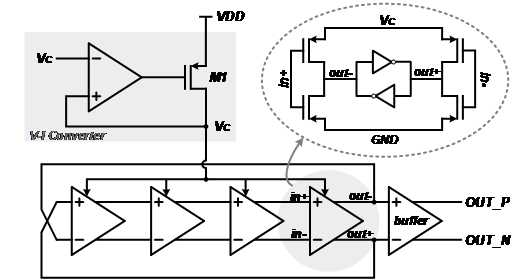## IV. PHASE NOISE ANALYSIS

Fig. 12 shows phase domain models for the four types of PLL for phase noise analysis [17,18]. The noise in a PLL mainly originates from the reference crystal oscillator, the RVCO, the LF, and the PFD/CP. In the RSPLL and SSPLL, the fine loop is only used for noise modeling. The LF transimpedance and the RVCO gain are denoted as F(s) and K$_{RVCO}$, respectively.

##### Fig. 12. Phase domain models for noise analysis: (a) NPLL; (b) MDLL; (c) SSPLL; (d) RSPLL.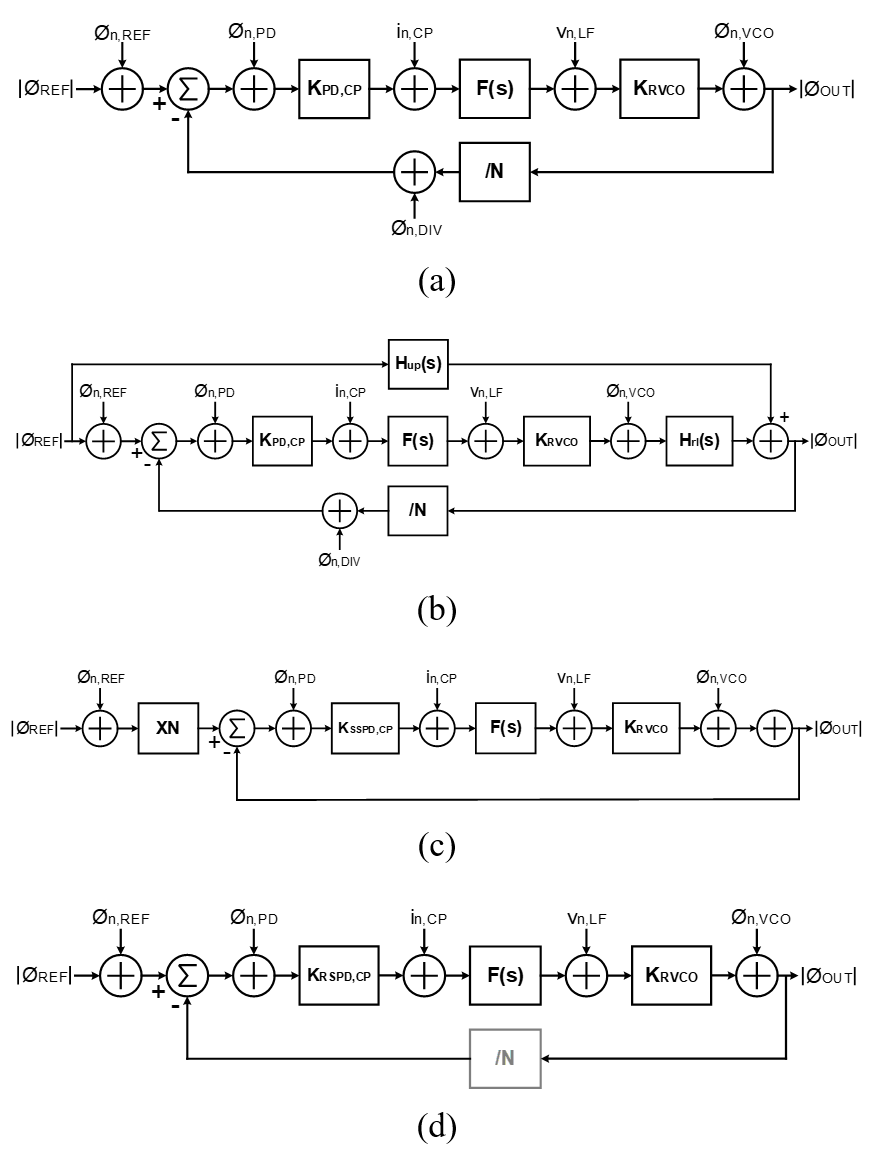### 1. NPLL

The noise analysis for the NPLL is based on Fig. 12(a). The open-loop transfer function for the NPLL (H$_{O,N}$) is

##### (1)
$H_{0,N}=\frac{K_{D,N}\cdot F\left(s\right)\cdot K_{RVCO}}{s}$

K$_{D,N}$is the PFD/CP gain of the NPLL. The noise power spectrum in the output of the NPLL from the reference crystal oscillator is

##### (2)
$S_{\mathrm{REF},\mathrm{n},\text{NPLL}}^{\mathrm{out}}=\mathrm{S}_{REF,n,NPLL}\left| \frac{\mathrm{H}_{0,\mathrm{N}}/\mathrm{N}}{1+\mathrm{H}_{0,\mathrm{N}}/\mathrm{N}}\right| ^{2}\mathrm{N}^{2}$

The reference noise is low-pass filtered and amplified by N$^{2}$ due to the dividing value of N. The PFD/CP gain (K$_{D,N}$) is modeled by I$_{CP}$/2${\pi}$ in the NPLL. The noise power spectrum in the output of the NPLL due to PFD/CP noise is calculated by

##### (3)
$S_{\mathrm{D},\mathrm{n},\text{NPLL}}^{\mathrm{out}}=\mathrm{S}_{\mathrm{D},\mathrm{n},\text{NPLL}}\left| \frac{\mathrm{H}_{0,\mathrm{N}}/\mathrm{N}}{1+\mathrm{H}_{0,\mathrm{N}}/\mathrm{N}}\cdot \frac{1}{\mathrm{K}_{\mathrm{D},\mathrm{N}}}\right| ^{2}\mathrm{N}^{2}$

The phase noise contribution of the PFD/CP becomes smaller with the PFD/CP gain than does the phase noise from the reference.

The corresponding noise spectra must be added to obtain the overall phase noise spectrum. The calculated output phase noise is presented in Fig. 13. The contributors to the phase noise are the reference crystal oscillator, the PFD/CP, and the VCO. The NPLL noise power spectrum at offsets below 1 kHz are dominated by the reference and CP noise, while higher offsets are dominated by VCO phase noise.

### 2. MDLL

The noise analysis for the MDLL is based on Fig. 12(b). H$_{up}$ represents the effect of reference selection, and H$_{rl}$ represents the oscillator’s phase realignment transfer function . H$_{up}$and H$_{rl}$ are defined as follows:

##### (4)
$H_{up}\left(s\right)=\frac{N\beta }{1+\left(\beta -1\right)e^{-j\omega {T_{r}}}}e^{-j\omega {T_{r}}/2}\text{sinc}\left(\omega \frac{T_{r}}{2}\right) \\$
##### (5)
$H_{rl}\left(s\right)=1-\frac{\beta }{1+\left(\beta -1\right)e^{-j\omega {T_{r}}}}e^{-\frac{j\omega T_{r}}{2}}\text{sinc}\left(\omega \frac{T_{r}}{2}\right)$

$\beta$ is the realignment coefficient. The open-loop transfer function of the MDLL is

##### (6)
$H_{o,M}=\frac{K_{D,M}\cdot F\left(s\right)\cdot K_{RVCO}\cdot H_{rl}}{s}$

K$_{D,M}$ is the PFD/CP gain for the MDLL. The noise power spectrum in the output of the MDLL from the reference crystal oscillator is

##### (7)
$S_{\mathrm{REF},\mathrm{n},\text{MDLL}}^{\mathrm{out}}=\mathrm{S}_{REF,n,MDLL}\left| \frac{\mathrm{H}_{0,\mathrm{M}}/\mathrm{N}}{1+\mathrm{H}_{0,\mathrm{M}}/\mathrm{N}}\cdot \left(1+H_{up}\right)\right| ^{2}\mathrm{N}^{2}$

H$_{up}$affects the reference noise power spectrum in the output. K$_{D,M}$ is the same as K$_{D,N}$ because they use the same PFD/CP. The noise power spectrum in the output from the PFD/CP is

##### (8)
$S_{\mathrm{D},\mathrm{n},\text{MDLL}}^{\mathrm{out}}=\mathrm{S}_{\mathrm{D},\mathrm{n},\text{MDLL}}\left| \frac{\mathrm{H}_{0,\mathrm{M}}/\mathrm{N}}{1+\mathrm{H}_{0,\mathrm{M}}/\mathrm{N}}\cdot \frac{1}{\mathrm{K}_{\mathrm{D},\mathrm{M}}}\right| ^{2}\mathrm{N}^{2}$

The calculated output phase noise for the MDLL is displayed in Fig. 13. Reference noise dominates below an offset frequency of 2 kHz but, above 2 kHz, VCO phase noise dominates. In the NPLL, jitter generated in the VCO continuously accumulates. However, in the MDLL, by replacing the rising edge of FOUT with the rising edge of FREF, the accumulated jitter is removed. The calculated phase noise for the MDLL exhibits an improvement of about 6.3 dB over the NPLL at an offset frequency of 20 kHz. The reference noise directly affects the noise spectrum in the output periodically, inducing reference spur.

##### Fig. 13. Calculated PLL output phase noise.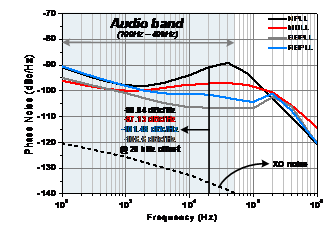### 3. SSPLL

A phase domain model for the SSPLL is shown in Fig. 12(c). An important difference from the phase domain models of the previous PLLs is that there is no N value in the feedback loop. The SSPD/SSCP can obtain a high gain. The gain of SSPD/SSCP is

##### (9)
$K_{D,SS}=2A_{VCO}\cdot \frac{\tau _{PUL}}{T_{ref}}\cdot g_{m,SS}$

where A$_{VCO}$ is the amplitude of FOUT, ${\tau}$$_{\mathrm{PUL}} is the pulse width of PUL, and g_{\mathrm{m,SS}} represents the single MOS transistor in M1 and M2 in the SSCP. The open-loop transfer function for the SSPLL is ##### (10) H_{0,SS}=\frac{K_{D,SS}\cdot F\left(s\right)\cdot K_{RVCO}}{s} The noise power spectrum in the output of the SSPLL from the reference crystal oscillator is ##### (11) S_{\mathrm{REF},\mathrm{n},\text{SSPLL}}^{\mathrm{out}}=\mathrm{S}_{REF,n,SSPLL}\left| \frac{\mathrm{H}_{0,\mathrm{SS}}}{1+\mathrm{H}_{0,\mathrm{SS}}}\right| ^{2}\mathrm{N}^{2} The SSPD/SSCP noise power spectrum in the output is ##### (12) S_{\mathrm{D},\mathrm{n},\text{SSPLL}}^{\mathrm{out}}=\mathrm{S}_{\mathrm{D},\mathrm{n},\text{SSPLL}}\left| \frac{\mathrm{H}_{0,\mathrm{SS}}}{1+\mathrm{H}_{0,\mathrm{SS}}}\cdot \frac{1}{\mathrm{K}_{\mathrm{D},\mathrm{SS}}}\right| ^{2} In the SSPLL, except reference noise, the phase noise contributions of the noise sources are not multiplied by N^{2}. The calculated output phase noise is summarized in Fig. 13. Reference noise dominates below an offset frequency of 10 kHz, but the VCO phase noise is dominant above 10 kHz. The calculated phase noise for the SSPLL exhibits an improvement of approximately 15.8 dB compared to the NPLL at an offset frequency of 20 kHz. ### 4. RSPLL A phase domain model for the RSPLL fine loop is shown in Fig. 12(d). Like the SSPLL, the RSPLL is a dividerless PLL, but it has the virtual dividing value N in the feedback loop. Unlike the tri-state PFD/CP, the RSPD/RSCP can generate a high gain, which is calculated as follows: ##### (13) K_{D,RS}=2\frac{T_{ref}\cdot A_{ref}}{2\pi \cdot t_{rising}}\cdot \frac{\tau _{PUL}}{T_{ref}}\cdot g_{m,RS} where A_{REF} is the amplitude of FREF, {\tau}$$_{\mathrm{PUL}}$ is the pulse width of PUL, t$_{rising}$ is the rising time of FREF, and g$_{\mathrm{m,RS}}$ is for the single MOS transistor in M1 and M2 in the RSCP.

The noise power spectrum in the output of the RSPLL from the reference crystal oscillator is

##### (14)
$S_{\mathrm{REF},\mathrm{n},\text{RSPLL}}^{\mathrm{out}}=\mathrm{S}_{REF,\mathrm{n},RSPLL}\left| \frac{\mathrm{H}_{0,\mathrm{RS}}/\mathrm{N}}{1+\mathrm{H}_{0,\mathrm{RS}}/\mathrm{N}}\right| ^{2}\mathrm{N}^{2}$

The RSPD/RSCP noise spectrum in the output is

##### (15)
$S_{\mathrm{D},\mathrm{n},\text{RSPLL}}^{\mathrm{out}}=\mathrm{S}_{\mathrm{D},\mathrm{n},\text{RSPLL}}\left| \frac{\mathrm{H}_{0,\mathrm{RS}}/\mathrm{N}}{1+\mathrm{H}_{0,\mathrm{RS}}/\mathrm{N}}\cdot \frac{1}{\mathrm{K}_{\mathrm{D},\mathrm{RS}}}\right| ^{2}\mathrm{N}^{2}$

Due to the virtual N value, the effect of N on the phase noise is not eliminated even though there is no N-divider in the fine loop. The RSPLL cannot take advantage of a dividerless PLL to the same extent as the SSPLL. However, a large K$_{D,RS}$ suppresses the output phase noise contribution of the RSPD/RSCP. The RSPLL can take advantage of the high-gain SPD in terms of phase noise when compared to the NPLL.

Reference noise and the RSPD/RSCP dominate below an offset frequency of 4 kHz, but the VCO phase noise is dominant above 4 kHz (Fig. 13). At an offset frequency of 20 kHz, the calculated phase noise improves by around 10.6 dB compared with the NPLL.

## V. MEASUREMENT RESULTS

Chips for the PLLs are fabricated using a Samsung 0.13-${\mu}$m CMOS process, and microphotographs are presented in Fig. 14. The chip area (including pads) is 864 ${\mu}$m $\times$ 967 ${\mu}$m for each PLL. The MDLL has the smallest core size of 364 ${\mu}$m $\times$ 320 ${\mu}$m, while the RSPLL has the largest (567 ${\mu}$m $\times$ 329 ${\mu}$m). The NPLL, MDLL, SSPLL, and RSPLL consume 1.82, 1.35, 1.43, and 1.64 mW, respectively, from a 1.5-V power supply. The PLLs are measured using the printed circuit board (PCB) shown in Fig. 15. The measured phase noise and spur performance at an output frequency of 24.576 MHz are presented in Fig. 16 and 17. The frequency of the VCO is divided by 4 at the post divider and by 64 at the N-divider to produce an output frequency of a 24.576 MHz. The reference frequency is 1.536 MHz, which originates from the external crystal oscillator.

The integrated jitter from 100 Hz to 40 kHz for the NPLL, MDLL, SSPLL, and RSPLL are 45.19, 24.05, 8.09, and 16.85 ps, respectively, while noise analysis predicted values of 49.66, 24.51, 9.61, and 15.79 ps respectively. The in-band phase noise at an offset of 20~kHz is -92.43, -97.45, -108.36, and -101.81 dBc/Hz,

which is similar to the expected -90.84, -97.13, -106.6, and -101.48 dBc/Hz (Fig. 16). The difference between the calculated and measured values at a lower offset is due to the effect of reference crystal oscillator phase noise, and the difference at a higher offset represents the effect of VCO phase noise. The measured reference spur is -38.74, -33.33, -41.77, and -40.93 dBc. The reference spur for the MDLL is the largest (Fig. 17). Table 1 summarizes the measured RMS jitter, reference spur, and figure-of-merit (FoM) for the PLLs.

##### Fig. 14. Chip microphotographs of the (a) NPLL; (b) MDLL; (c) SSPLL; (d) RSPLL.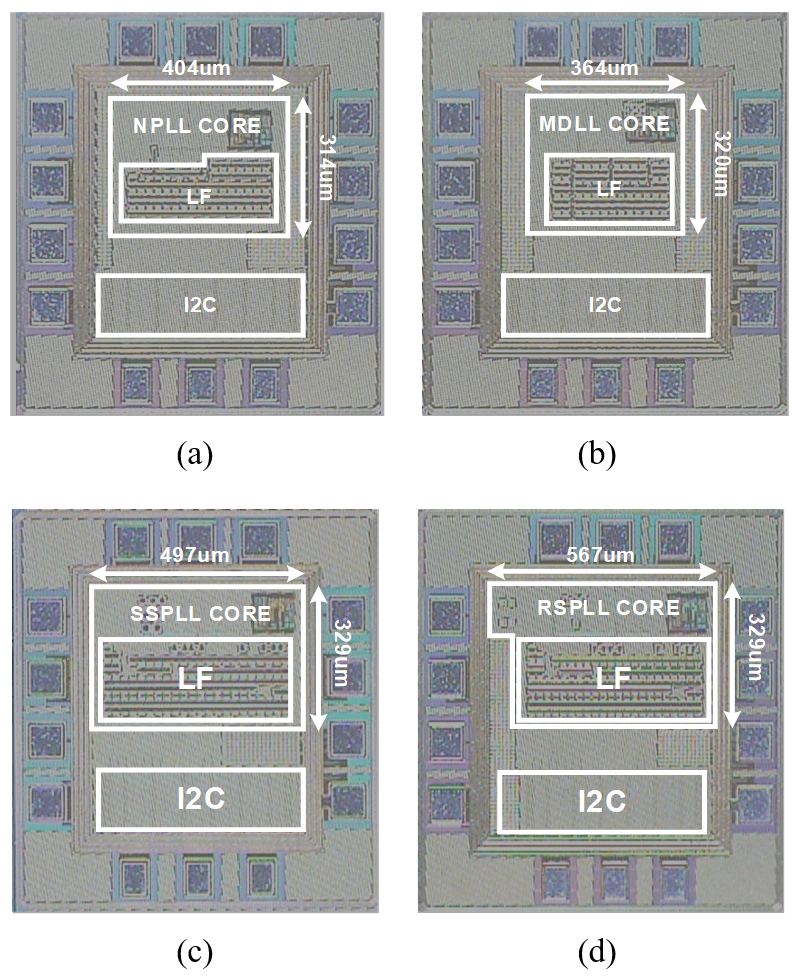##### Fig. 15. PCB used for measurement.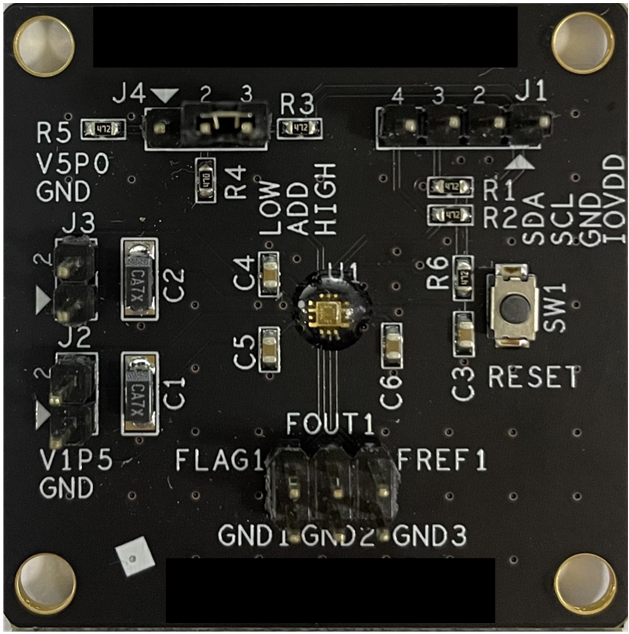##### Fig. 16. Measured PLL output phase noise.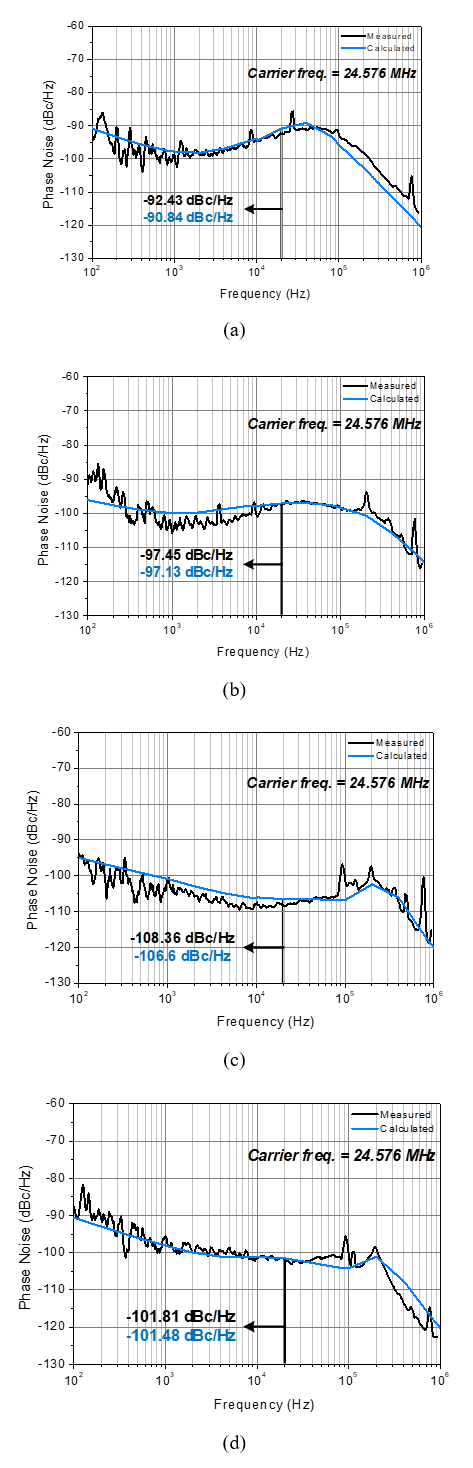##### Fig. 17. Measured PLL output spectra for the (a) NPLL; (b) MDLL; (c) SSPLL; (d) RSPLL.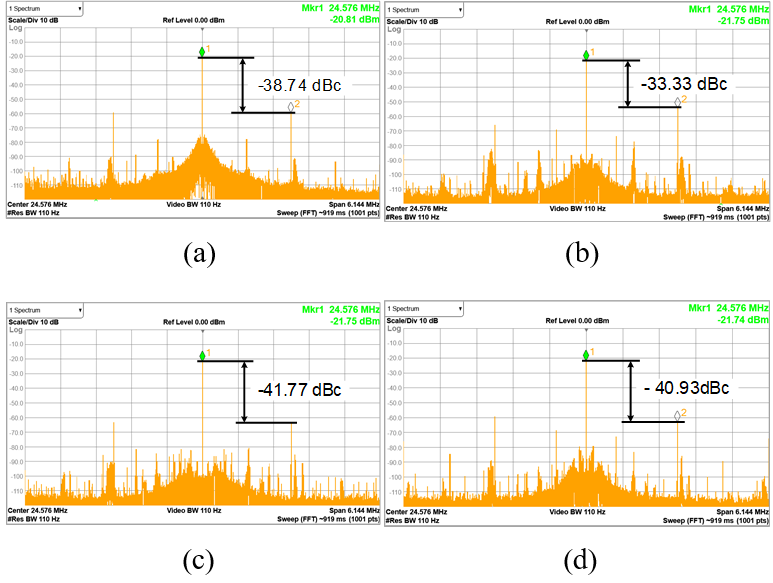##### Table 1. Performance summary (measurements)
 Jitter [ ps$_{\mathrm{rms}}$] Spur[dBc] FoM[dB] NPLL 45.19 -38.74 -204.3 MDLL 24.05 -33.33 -211.07 SSPLL 8.09 -41.77 -220.29 RSPLL 16.85 -40.93 -213.32

## VI. CONCLUSIONS

In this paper, four low-noise and low-power PLLs for mobile audio applications are investigated by conducting noise analysis and comparing the results to measurements taken from fabricated chips. The noise characteristics are well expected in the noise analysis. The power consumptions for the NPLL, MDLL, SSPLL, and RSPLL are 1.82, 1.35, 1.43, and 1.64 mW, respectively, from a 1.5-V power supply. The measured rms output jitter from 100 Hz to 40 kHz for the NPLL, MDLL, SSPLL and RSPLL are 45.19, 24.05, 8.09, and 16.85 ps, respectively. The measured in-band phase noise is -92.43, -97.45, -108.36, and -101.81 dBc/Hz at an offset of 20 kHz. The NPLL, MDLL, SSPLL, and RSPLL achieve an FoM of -204.3, -211.07, -220.29, and -213.32 dB.

In the case of MDLL, compared with NPLL as a reference, it can be confirmed that the jitter is improved by removing accumulated jitter due to reference injection. However, as in Eq. (7), the reference spur appears large. In Fig. 17(b), the reference spur appears as large as -33.33 dBc. In the case of SSPLL, except for the phase noise by reference, the N factor disappears from the noise equation. Therefore, the phase noise of the SSPLL is significantly improved compared to the NPLL. Reference noise affected by N factor has a dominant value up to 1 kHz, which can improve phase noise through SSPD/SSCP gain control. In the case of RSPLL, even though there is no N divider, N is inevitably added to the transfer function due to the sampling direction. It can be confirmed that the N value is in the same position as the NPLL in the noise equations. However, there is an improvement in phase noise in in-band and out-band due to gain control of RSPD/RSCP. There is a problem in that it is difficult to increase the gain compared to SSPD/SSCP.

## ACKNOWLEDGMENTS

This research was supported by the Technology Innovation Program (or Industrial Strategic technology development program, 1415178260, Envelope Tracking, Synchronous Boost Converter, Smart Power Amplifier, Power Management, Mobile SoC), and Chung-Ang University Graduate Research Scholarship in 2021. The EDA Tool was supported by the IC Design Education Center.

## References

1
2015, Inter-IC Sound Bus (I2S) 2.70 component datasheet, Infineon, Neubiberg, Germany2
Crawford J. A., 1994, Frequency Synthesizer Design Handbook, Boston, MA: Artech House3
Santiccioli Alessio, et al , Nov. 2019, A 1.6-to-3.0-GHz Fractional-N MDLL With a Digital-to-Time Converter Range-Reduction Technique Achieving 397-fs Jitter at 2.5-mW Power, IEEE Journal of Solid-State Circuits, Vol. 54, No. 11, pp. 3149-31604
Santiccioli Alessio, et al , Dec. 2020, A 66-fs-rms jitter 12.8-to-15.2-GHz fractional-N bang-bang PLL with digital frequency-error recovery for fast locking, IEEE Journal of Solid-State Circuits, Vol. 55, No. 12, pp. 3349-33615
Zhang Zhao, Zhu Guang, Patrick Yue C., Jun. 2020, A 0.65-V 12-16-GHz sub-sampling PLL with 56.4-fs rms integrated jitter and${-}$ 256.4-dB FoM, IEEE Journal of Solid-State Circuits, Vol. 55, No. 6, pp. 1665-16836
Elkholy Ahmed, et al , Jun. 2018, Low-jitter multi-output all-digital clock generator using DTC-based open loop fractional dividers, IEEE Journal of Solid-State Circuits, Vol. 53, No. 6, pp. 1806-18177
Farjad-Rad Ramin, et al , Dec. 2002, A low-power multiplying DLL for low-jitter multigigahertz clock generation in highly integrated digital chips, IEEE Journal of Solid-State Circuits, Vol. 37, No. 12, pp. 1804-18128
Mercandelli Mario, et al , Feb. 2020, A 12.5-GHz fractional-N type-I sampling PLL achieving 58-fs integrated jitter, IEEE Int. Solid-State Circuits Conf. (ISSCC) Dig. Tech. Papers, pp. 274-2769
Kim Juyeop, et al , Dec. 2019, An ultra-low-jitter, mmW-band frequency synthesizer based on digital subsampling PLL using optimally spaced voltage comparators, IEEE Journal of Solid-State Circuits, Vol. 54, No. 12, pp. 3466-347710
Liao Dongyi, et al , Mar, 2018, A 2.4-GHz 16-phase sub-sampling fractional-N PLL with robust soft loop switching, IEEE Journal of Solid-State Circuits, Vol. 53, No. 3, pp. 715-72711
Seol Ji-Hwan, et al , Oct. 2021, Reference oversampling PLL achieving${-}$ 256-dB FoM and${-}$ 78-dBc reference spur, IEEE Journal of Solid-State Circuits, Vol. 56, No. 10, pp. 2993-300712
Liao Dongyi, Dai Fa Foster , Mar. 2021, A fractional-N reference sampling PLL with linear sampler and CDAC based fractional spur cancellation, IEEE Journal of Solid-State Circuits, Vol. 56, No. 3, pp. 694-70413
Gao Xiang, et al , Dec. 2009, A low noise sub-sampling PLL in which divider noise is eliminated and PD/CP noise is not multiplied by N$^{2}$, IEEE Journal of Solid-State Circuits, Vol. 44, No. 12, pp. 3253-326314
Sharma J., Krishnaswamy H., May 2019, A 2.4-GHz reference-sampling phase-locked loop that simultaneously achieves low-noise and low-spur performance, IEEE Journal of Solid-State Circuits, Vol. 54, No. 5, pp. 1407-142415
Raj Mayank, et al , Jun. 2017, A 164fsrms 9-to-18GHz sampling phase detector based PLL with in-band noise suppression and robust frequency acquisition in 16nm FinFET, 2017 Symposium on VLSI Circuits. IEEE, pp. 182-18316
Dai Liang, Harjani Ramesh , 2001, A low-phase-noise CMOS ring oscillator with differential control and quadrature outputs, Proceedings 14th Annual IEEE International ASIC/SOC Conference (IEEE Cat. No. 01TH8558). IEEE, pp. 113-11817
Elshazly Amr, et al , Jun. 2013, Clock multiplication techniques using digital multiplying delay-locked loops, IEEE Journal of Solid-State Circuits, Vol. 48, No. 6, pp. 1416-142818
Sharma J., 2018, CMOS signal synthesizers for emerging RF-to-optical applications, Columbia University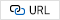19
Elshazly Amr, et al , Jun. 2013, Clock multiplication techniques using digital multiplying delay-locked loops, IEEE Journal of Solid-State Circuits, Vol. 48, No. 6, pp. 1416-1428Yujin Kyung received her B.S. degree from the Department of Electrical and Electronics Engi-neering, Chung-Ang University, Seoul, Korea in 2021. She is currently pursuing an M.S. degree in the Department of Electrical and Electronics Engineering, Chung-Ang University, Seoul, Korea. Her research interests include analog circuit design and phase-locked loop design.

Gwang Sub Kim received the B.S. and M.S. degrees in electrical engi-neering from Chung-Ang University, Seoul, South Korea, in 2018 and 2020 respectively. He is working towards his Ph.D. at the School of Electrical Engineering at Chung-Ang University. His research interest is the design of mixed-mode signal IC design including RF. He mainly studies VCO and PLL, which are frequency synthesizers used in wireless communication systems.

Donghyun Baek received his B.S., M.S., and Ph.D. degree in the Department of Electrical Engineering of the Korea Advanced Institute of Science and Technology (KAIST), Daejeon, Korea, in 1996, 1998, and 2003, respectively. From 2003 to 2007, he was with the System LSI Division in Samsung Electronics Company, Kiheung, Korea, where he designed mobile and ISTB-T and led the CMOS power amplifier project for handsets. In 2007, he joined the school of Electrical Engineering, Chung-Ang University, Seoul, Korea, where he is currently a professor. He is a life member of the IEIE and a senior member of the IEEE. His research interests include analog, RF, and mixed-mode circuit designs for mobile system-on-chip, radar-on-chip, and sensor-on-chip integrated circuits.